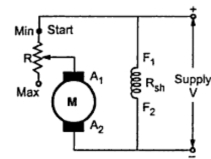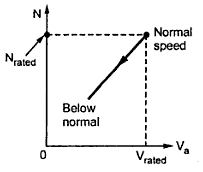### Armature Voltage Control Method or Rheostatic Control

Speed Control of D.C. Shunt Motor (Part2)

2. Armature Voltage Control Method or Rheostatic Control
The speed is directly proportional to the voltage applied across the armature. As the supply voltage is normally constant, the voltage across the armature can be controlled by adding a variable resistance in series with the armature as shown in the Fig. 1.Fig. 1 Rheostat control of shunt motor

The field winding is excited by the normal voltage hence Ish is rated and constant in this method. Initially the reheostat position is minimum and rated voltage gets applied across the armature. So speed is also rated. For a given load, armature current is fixed. So when extra resistance is added in the armature circuit, Ia remains same and there is voltage drop across the resistance added (Ia R). Hence voltage across the armature decreases, decreasing the speed below normal value. By varying this extra resistance, various speeds below rated value can be obtained.
So far a constant load torque, the speed is directly proportional to the voltage across the armature. The relationship between speed and voltage across the armature is shown in the Fig. 2.Fig. 2  N Vs voltage across armature
1.1 Potential Divider Control
The main disadvantages of the above method is, the speed up to zero is not possible as it requires a large rheostat in series with the armature which is practically impossible. If speed control from zero to the rated speed is required, by rheostatic method then voltage across the armature can be varied by connecting rheostat in a potential divider arrangement as shown in the Fig. 3.Fig. 3  Potential divider arrangement

When the variable rheostat position is at 'start' point shown, voltage across the armature is zero and hence speed is zero. As rheostat is moved towards 'maximum' point shown, the voltage across the armature increases, increasing the speed. At maximum point the voltage is maximum i.e. rated hence maximum speed possible is rated speed. The relationship is shown in the Fig. 4.Fig. 4  N  Vs  V

When the voltage across the armature starts increasing, as long as motor does not overcome inertial and frictional torque, the speed of the motor remains zero. The motor requires some voltage to start hence the graph of voltage and the speed does not pass through the origin as shown in the Fig.4.
1. Easy and smooth speed control below normal is possible.
2. In potential divider arrangement, rheostat can be used as a starrer.
1. As the entire armature current passes through the external resistance, there are tremendous power losses.
2. As armature current is ore than field current, rheostat required is of large size and capacity.
3. Speed above rated is not possible by this method.
4. Due to large power losses, the method is expensive, wasteful and less efficient.
5. The method needs expensive heat dissipation arrangements.

Related Articles :
Significance of Back E.M.F.